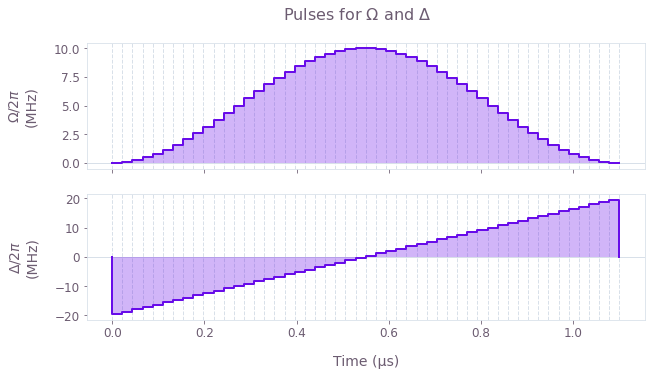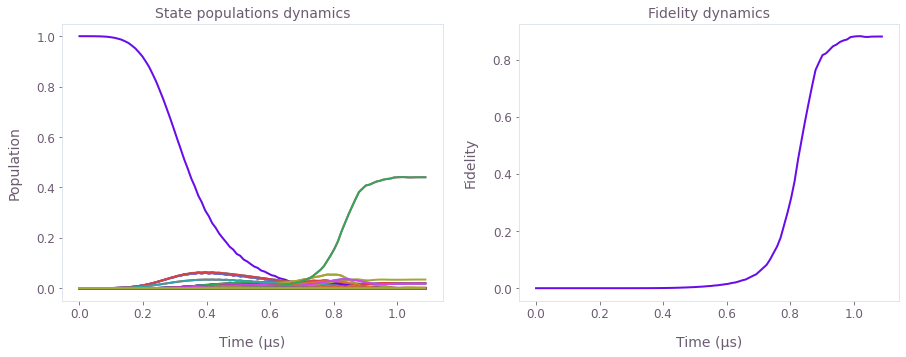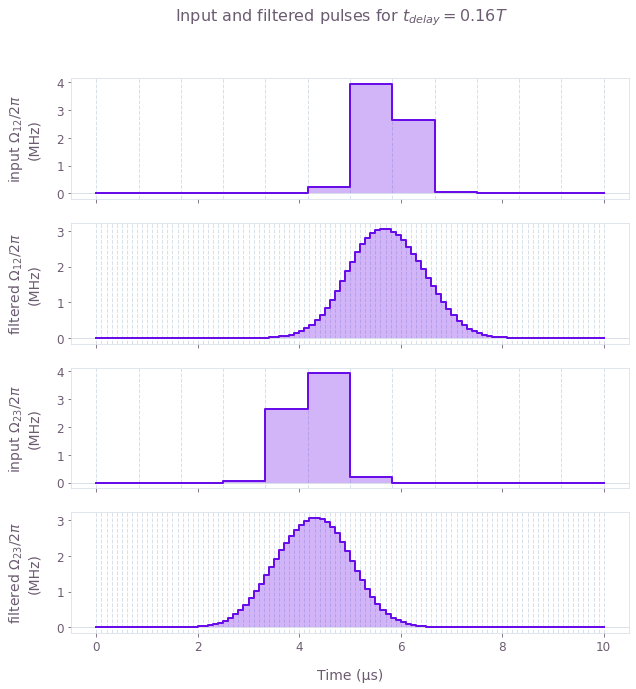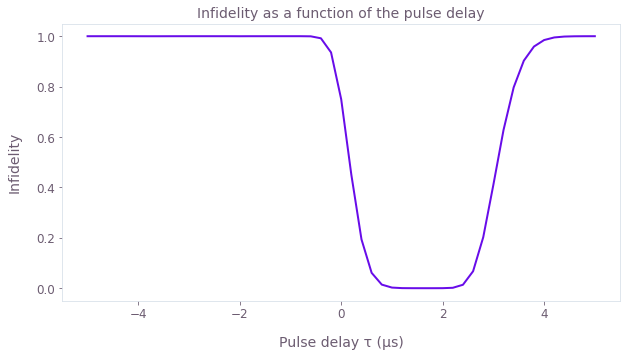•Boulder Opal
• User guides
• Simulate quantum dynamics for noiseless systems using graphs

# How to simulate quantum dynamics for noiseless systems using graphs

Simulate the dynamics of closed quantum systems

Boulder Opal enables you to simulate the dynamics of quantum systems that are subject to complex deterministic Hamiltonians. Simulation of quantum dynamics can benefit from graph-based calculation when elements of the computation undergo transformations linked to other nodes. For instance, considering simulation of a quantum system subject to a distorted control pulse, we can combine the source of distortion into the computational graph in order to flexibly simulate the composite system.

In this notebook we show how to use Boulder Opal to perform simulations of multilevel systems with complex coupling mechanisms using graph-based simulation. This approach is preferable when a simulation becomes part of a larger computation where flexibility is prioritized.

## Summary workflow

Here we show how to use Boulder Opal to create and execute a graph made up of nodes (corresponding to the different steps in the simulation) that represent functions of previous nodes (or of constant values). Please see the currently available primitive nodes.

### 1. Define computational graph

To describe the dynamics of a noiseless quantum system with Boulder Opal, start by setting up a graph object as described in the How to represent quantum systems using graphs user guide.

### 2. Set up quantum system

Within the graph, define the piecewise-constant (PWC) system Hamiltonian. This is done by combining time-dependent signals with the corresponding operators for each term in the Hamiltonian. Signals are created using the graph.pwc_signal operation, while the operators are represented by constant matrices.

### 3. Create simulation node

Define a simulation node using the graph.time_evolution_operators_pwc operation. This calculates the unitary operation corresponding to the evolution of the system at times defined by the array sample_times. From these unitaries, one can calculate the state, or any system observable, at the specified times.

### 4. Execute graph-based simulation

Execute the graph-based simulation by calling the qctrl.functions.calculate_graph function. After the simulation is successfully completed, you can extract the results explicitly defined in the output_node_names, as described in the How to represent quantum systems using graphs user guide.

The graph-based approach also offers the possibility of running multiple simulations with a single call to qctrl.functions.calculate_graph. For this, instead of defining a single Hamiltonian, you can define a batch of them, as examplified in the STIRAP example below.

## Example: Generate entangled states in atomic chains

In this example we show how to simulate the adiabatic dynamics of a multi-atomic system going from the ground state to an entangled state involving all atoms. We start by creating a graph with the qctrl.create_graph function and adding operations to it in order to simulate the dynamics of an atomic chain.

We consider a chain of $N=8$ atoms, each one modeled as a qubit, with $|0\rangle$ representing the ground state and $|1\rangle$ representing a Rydberg state. The total Hamiltonian of the system, as described by A. Omran et al., is given by

$$H = \frac{\Omega(t)}{2} \sum_{i=1}^N \sigma_x^{(i)} - \Delta(t) \sum_{i=1}^N n_i - \sum_{i=1}^N \delta_i n_i + \sum_{i<j}^N \frac{V}{\vert i-j \vert^6}n_i n_j ,$$

with $\sigma_x^{(i)} = \vert 0 \rangle \langle 1 \vert_i + \vert 1 \rangle \langle 0 \vert_i$, and $n_i = \vert 1\rangle\langle 1 \vert_i$. The fixed system parameters are the interaction strength between excited atoms, $V= 2\pi \times 24$ MHz, and the local energy shifts $\delta_i = - 2 \pi \times 4.5$ MHz for $i=1,N$ or 0 otherwise.

We consider the system to be initially in its ground state, $|\psi(t=0)\rangle = |00000000\rangle$ , and we aim to prepare the entangled target state $|\psi_\mathrm{target}\rangle = \frac{1}{\sqrt{2}} \Big( |01010101\rangle + |10101010\rangle \Big)$. To achieve this superposition, the effective coupling strength to the Rydberg state $\Omega(t)$ is modulated by a $\sin^2$ envelope while the detuning $\Delta(t)$ is linearly swept around zero, for a total time of $T = 1.1$ µs.

import matplotlib.pyplot as plt
import numpy as np
from qctrlvisualizer import get_qctrl_style, plot_controls

from qctrl import Qctrl

# Start a Boulder Opal session
qctrl = Qctrl()

plt.style.use(get_qctrl_style())
# Physical parameters of the systems
N_qubits = 8
interaction_strength = 24.0e6 * (2.0 * np.pi)  # Hz
local_shift = -4.5e6 * (2.0 * np.pi)  # Hz
omega_max = 10.0e6 * (2.0 * np.pi)  # Hz
delta_max = 20.0e6 * (2.0 * np.pi)  # Hz
total_duration = 1.1e-6
segments = 50
sample_times = np.linspace(0.0, total_duration, 100, endpoint=False)

# Create the data flow graph describing the system
graph = qctrl.create_graph()

# Coupling strength to the Rydberg state operator
sigma_x_sum = sum(
graph.pauli_kronecker_product([("X", k)], N_qubits) for k in range(N_qubits)
)

# Detuning operator
n_operator_list = [
0.5 * graph.pauli_kronecker_product([("I", k)], N_qubits)
- 0.5 * graph.pauli_kronecker_product([("Z", k)], N_qubits)
for k in range(N_qubits)
]
n_operator_sum = graph.sum(n_operator_list, 0)

# Local energy shift operator
local_shifts = np.zeros(N_qubits)
local_shifts = local_shift
local_shifts[-1] = local_shift
local_shift_operator = sum(shift * n for shift, n in zip(local_shifts, n_operator_list))

# Interaction operator
interaction_operator = 0
for idx1 in range(N_qubits):
for idx2 in range(idx1):
n1_n2 = n_operator_list[idx1] @ n_operator_list[idx2]
interaction_operator += (n1_n2) / np.abs(idx1 - idx2) ** 6
interaction_operator *= interaction_strength

# Initial state
initial_state = graph.fock_state(2**N_qubits, 0)

# Target GHZ state
idx_even = sum(2**n for n in range(0, N_qubits, 2))  # |0101...>
idx_odd = sum(2**n for n in range(1, N_qubits, 2))  # |1010...>
GHZ_state = np.zeros([2**N_qubits])
GHZ_state[[idx_even, idx_odd]] = 1.0 / np.sqrt(2.0)

# Signals for delta and omega
omega_signal = graph.signals.cosine_pulse_pwc(
duration=total_duration, segment_count=segments, amplitude=omega_max, name="omega"
)
delta_signal = graph.signals.linear_ramp_pwc(
duration=total_duration, segment_count=segments, end_value=delta_max, name="delta"
)

# Construct the Hamiltonian
omega_term = 0.5 * omega_signal * sigma_x_sum
delta_term = -delta_signal * n_operator_sum
constant_term = interaction_operator - local_shift_operator

hamiltonian = omega_term + delta_term + constant_term

# Calculate the evolution unitaries and evolve the initial state
unitaries = graph.time_evolution_operators_pwc(
hamiltonian=hamiltonian, sample_times=sample_times
)
evolved_states = unitaries @ initial_state[:, None]
evolved_states.name = "evolved_states"

# Project the evolved and target states to calculate the fidelity
fidelity = graph.abs(GHZ_state[None] @ evolved_states) ** 2
fidelity.name = "fidelity"

# Run simulation
graph_result = qctrl.functions.calculate_graph(
graph=graph, output_node_names=["omega", "delta", "evolved_states", "fidelity"]
)

# Plot the controls
plot_controls(
{
"$\\Omega$": graph_result.output["omega"],
"$\\Delta$": graph_result.output["delta"],
}
)
plt.suptitle("Pulses for $\\Omega$ and $\\Delta$")

# Retrieve and plot the evolved states and fidelity
evolved_states = graph_result.output["evolved_states"]["value"].squeeze()
fidelity = graph_result.output["fidelity"]["value"].squeeze()

fig, axs = plt.subplots(1, 2, figsize=(15, 5))
axs.plot(sample_times * 1e6, np.abs(evolved_states) ** 2)
axs.set_xlabel("Time (µs)")
axs.set_ylabel("Population")
axs.set_title("State populations dynamics")
axs.plot(sample_times * 1e6, fidelity)
axs.set_xlabel("Time (µs)")
axs.set_ylabel("Fidelity")
axs.set_title("Fidelity dynamics")
plt.show()
Your task calculate_graph (action_id="1599486") has started.## Example: Simulate the effect of pulse delay on STIRAP

In this example we show how to simulate the dynamics of a three-level system undergoing stimulated Raman adiabatic passage (STIRAP) when subjected to pump and Stokes pulses. If the pulses are applied in a counter-intuitive manner, that is, $\Omega_{23}(t)$ is applied before $\Omega_{12}(t)$ (or $t_\textrm{delay} >0$), the STIRAP pulse sequence will transfer the population in state $|1\rangle$ to state $|3\rangle$. In the calculation below, we will see the effect of $t_\textrm{delay}$ on the infidelity of the process. Moreover, we will assume that the input controls are very coarse, consisting of very few segments, but they are passed through a Gaussian filter before acting on the three-level system.

The Hamiltonian of the system is given by \begin{equation} H(t) = \frac{1}{2} \Omega_{12}(t) \Big(|1\rangle\langle 2| + |2\rangle\langle 1|\Big) + \frac{1}{2} \Omega_{23}(t) \Big(|2\rangle\langle 3| + |3\rangle\langle 2|\Big) , \end{equation} where the time-dependent Rabi rates for the pump and Stoke pulses are applied for $t\in(0,T)$ with a Gaussian profile: \begin{equation} \Omega_{12}(t) = \Omega_\max \exp\left(- \frac{\left(t - \frac{T}{2} - \frac{t_\textrm{delay}}{2}\right)^2}{2\sigma^2}\right) \\ \Omega_{23}(t) = \Omega_\max \exp\left(- \frac{\left(t - \frac{T}{2} + \frac{t_\textrm{delay}}{2}\right)^2}{2\sigma^2}\right) \end{equation} with a maximum amplitude $\Omega_\max$, a pulse width $\sigma$, and a delay between them of $t_\textrm{delay}$.

# Define matrices for the Hamiltonian operators
a_12 = np.array([[0, 1, 0], [1, 0, 0], [0, 0, 0]])
a_23 = np.array([[0, 0, 0], [0, 0, 1], [0, 1, 0]])

# Define the target unitary: state |1> goes to state |3>
target_operation = np.array([[0, 0, 0], [0, 0, 0], [1, 0, 0]])

# Define system parameters
omega_max = 2 * np.pi * 5e6  # Hz
total_duration = 10.0e-6  # s
sigma = 0.5e-6
gauss_filter_std = 0.5e-6
input_segment_count = 12
filtered_segment_count = 100

times = np.linspace(0.0, total_duration, input_segment_count)
durations = np.array([total_duration / input_segment_count] * input_segment_count)

Below we create a batch of input Gaussian pulses (for different values of $t_\textrm{delay}$), filter them, and use them to simulate the time evolution of the system. The batch of pulses allows the computations for all values of $t_\textrm{delay}$ to be performed in parallel when the graph is executed. In this case, the first dimension of the $\Omega$ pulse corresponds to the number of delay values in the batch (defined as 51 here) and the second dimension accounts for the time points sampled from the evolution (defined by input_segment_count = 12 in this example).

# Define values of t_delay
t_delay_values = np.linspace(-total_duration / 2, total_duration / 2, 51)

# Create batch of coarse input pulses
omega_12_pulse = omega_max * np.exp(
-0.5
* (times[None] - total_duration / 2.0 - t_delay_values[:, None] / 2.0) ** 2
/ sigma**2
)
omega_23_pulse = omega_max * np.exp(
-0.5
* (times[None] - total_duration / 2.0 + t_delay_values[:, None] / 2.0) ** 2
/ sigma**2
)

In this particular example, we are interested only in the infidelities of the operation for each time delay, which can be extracted directly from the graph.infidelity_stf operation.

# Create the data flow graph describing the system
graph = qctrl.create_graph()

# Create input signals
omega_12_signal = graph.pwc_signal(
values=omega_12_pulse, duration=total_duration, name="omega_12_signal"
)
omega_23_signal = graph.pwc_signal(
values=omega_23_pulse, duration=total_duration, name="omega_23_signal"
)

# Filter signals through a Gaussian filter
filtered_omega_12_signal = graph.convolve_pwc(
omega_12_signal, graph.gaussian_convolution_kernel(gauss_filter_std)
)
filtered_omega_23_signal = graph.convolve_pwc(
omega_23_signal, graph.gaussian_convolution_kernel(gauss_filter_std)
)

# Discretize filtered signals to output smoothed signals
sampled_filtered_pulse_12 = graph.discretize_stf(
stf=filtered_omega_12_signal,
duration=total_duration,
segment_count=filtered_segment_count,
name="filtered_omega_12_signal",
)
sampled_filtered_pulse_23 = graph.discretize_stf(
stf=filtered_omega_23_signal,
duration=total_duration,
segment_count=filtered_segment_count,
name="filtered_omega_23_signal",
)

# Create Hamiltonian terms
omega_12_term = filtered_omega_12_signal * a_12
omega_23_term = filtered_omega_23_signal * a_23

# Define the target operation
target = graph.target(operator=target_operation)

# Calculate the infidelities
infidelities = graph.infidelity_stf(
sample_times=np.linspace(0, total_duration, filtered_segment_count),
hamiltonian=omega_12_term + omega_23_term,
target=target,
name="infidelities",
)

We now perform the simulation and retrieve the returned control infidelities. We then plot both the infidelities as well as a pair of the input/filtered pulses simulated.

# Run simulation
graph_result = qctrl.functions.calculate_graph(
graph=graph,
output_node_names=[
"infidelities",
"omega_12_signal",
"omega_23_signal",
"filtered_omega_12_signal",
"filtered_omega_23_signal",
],
)

# Plot the input/filtered pulses
plot_controls(
{
"input $\\Omega_{12}$": graph_result.output["omega_12_signal"],
"filtered $\\Omega_{12}$": graph_result.output["filtered_omega_12_signal"],
"input $\\Omega_{23}$": graph_result.output["omega_23_signal"],
"filtered $\\Omega_{23}$": graph_result.output["filtered_omega_23_signal"],
}
)
plt.suptitle("Input and filtered pulses for $t_{delay} = 0.16 T$")

# Plot the infidelities obtained as a function of the pulse delay
plt.figure()
plt.plot(t_delay_values / 1e-6, graph_result.output["infidelities"]["value"])
plt.xlabel("Pulse delay τ (µs)")
plt.ylabel("Infidelity")
plt.title("Infidelity as a function of the pulse delay")
plt.show()
Your task calculate_graph (action_id="1599493") has completed.Notice that the infidelity only goes to 0 when $t_\textrm{delay} > 0$, but if the two pulses are too separated the infidelity increases again.

This notebook was run using the following package versions. It should also be compatible with newer versions of the Q-CTRL Python package.

PackageVersion
Python3.10.8
matplotlib3.6.3
numpy1.24.1
scipy1.10.0
qctrl20.1.1
qctrl-commons17.7.0
boulder-opal-toolkits2.0.0-beta.3
qctrl-visualizer4.4.0